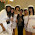## Pic## Wednesday, August 1, 2012

### Pop Quizzes and Probability

Here's a fun probability problem that worked pretty well in class, and is pretty easy to pull off:

1.  Tell everyone to clear their desks. It's a pop quiz. Use your serious face.
2.  Hand them this:

3.  Read the questions out loud. It gets kind of fun.
4.  What's the question that everyone wants to know? In my classes, it was "Wait, did I pass?" It was a smooth transition from that question to the question I asked: "Well, what are the chances that you passed?"

Then we're in familiar territory. Take guesses, write them on the board. Take ideas from the whole group. Circulate, offer suggestions, ask questions, give hints as you see fit.

The kids won't let you forget to read out the answers before the end of class.

Postscript

There was a pretty interesting design question that came up as I was planning this activity: what should the quiz questions be? In order to get random guessing you just need a set of problems that kids couldn't possibly know the answers to. Here were some alternatives that I came up with when planning this problem:

In the end,  I wanted a set of questions that kids could think -- just for a second -- that I actually expected them to know. So I went with some Linear Algebra pulled from a test I took in college. But something else might work for your students.

1.And what are the answers to this linear algebra quiz? This looks fun.

2.It's so sad, but I've forgotten so much of Linear Algebra. I keep on telling myself that I want to sit down and do some problems every week, but that's not happening in the near future. Incidentally, what Linear Algebra textbooks do people like? I liked Linear Algebra Done Right in college, but I have no perspective on the matter.

3.Nice approach, right up my alley. I'd prefer multiple-choice questions, however, because there is something about 1/2 that's too simple to really point to the overall pattern of what's going on, if you see what I mean? Where do you go from here? I'd say this leads nicely into expected values of probability distributions, maybe even into binomial distribution.

1.This problem is densely connected to binomial distribution, Pascal's triangle, and more complicated binomial probability problems. This is coming somewhat near the end of a probability unit (i.e. this is, more or less, what we're heading towards) but it's a smooth move to statistics and other probability distributions from here.

4.I love the Peanuts reference. (I hope it's a Peanuts reference.)

1.Oh, it's a Peanuts reference.

5.We do this in our book series (CME Project, Precalculus, Investigation 7B), with a 20-question quiz and 5 possible answers to each question. It's done as an opener to a unit where students learn the binomial probability rules.

One thing you might try is giving this quiz to a LOT of people, then building the distribution of results. This gives you a chance to talk about experimental versus theoretical probability, and the shape of the distribution can point you toward talking about the normal distribution and the Central Limit Theorem.

Cool!
- Bowen